# SSAT Elementary Level Math : How to find the whole from the part

## Example Questions

1 2 31 32 33 34 35 36 37 39 Next →

### Example Question #131 : Read And Write Numbers To 1000 By Numerals, Number Names, And Expanded Form: Ccss.Math.Content.2.Nbt.A.3

What number is six hundred twelve?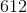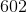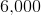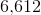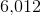Explanation:in word form is six hundred twelve.

### Example Question #2504 : Isee Lower Level (Grades 5 6) Quantitative Reasoning

What number is three hundred five?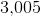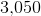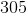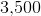Explanation:in word form is three hundred five.

### Example Question #2505 : Isee Lower Level (Grades 5 6) Quantitative Reasoning

What number is four hundred forty-seven?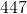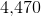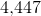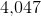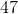Explanation:in word form is four hundred forty-seven.

### Example Question #12 : Read And Write Numbers To 1000 By Numerals, Number Names, And Expanded Form: Ccss.Math.Content.2.Nbt.A.3

The following number is written in expanded form. What is the number as a numeral?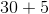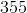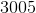Explanation:

When a number is in expanded form, you add the numbers together to find the numeral.

The numeral form of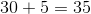.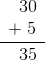### Example Question #73 : Read, Write, And Compare Multi Digit Numbers Using Base Ten Numerals, Number Names, And Expanded Form: Ccss.Math.Content.4.Nbt.A.2

Fill in the blank with the correct symbol.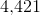__________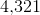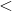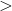Explanation:is grater thanbecause of the digit in the hundreds place.is greater than. The correct symbol isbecause that means greater than.

### Example Question #2502 : Isee Lower Level (Grades 5 6) Quantitative Reasoning

What number is five hundred thirty-three?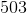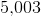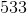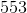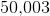in word form is five hundred thirty-three.